# loopsyn

Loop-shaping controller design with tradeoff between performance and robustness

## Syntax

``[K,CL,gamma,info] = loopsyn(G,Gd)``
``[K,CL,gamma,info] = loopsyn(G,Gd,alpha)``
``[K,CL,gamma,info] = loopsyn(G,Gd,alpha,ord)``

## Description

`loopsyn` balances performance and robustness by blending two loop-shaping methods.

• Mixed-sensitivity design (`mixsyn`), which tends to optimize performance and decoupling at the expense of robustness

• The Glover-McFarlane method (`ncfsyn`), which maximizes robustness to plant uncertainty

You can adjust the tradeoff between performance and robustness to obtain satisfactory time-domain responses while avoiding fragile designs with plant inversion or flexible mode cancellation.

example

````[K,CL,gamma,info] = loopsyn(G,Gd)` computes a stabilizing controller `K` that shapes the open-loop response `G*K` to approximately match the specified loop shape `Gd`. The mixed-sensitivity performance `gamma` indicates the closeness of the match. `loopsyn` tries to minimize `gamma`, subject to the constraint that the robustness with `K` (as measured by `ncfmargin`) is no worse than half the maximum achievable robustness. The function also returns the closed-loop transfer function `CL` and a structure `info` containing further information about the controller synthesis.```

example

````[K,CL,gamma,info] = loopsyn(G,Gd,alpha)` explicitly specifies the tradeoff between performance and robustness with parameter `alpha` in the interval [0,1]. Within this interval, smaller `alpha` favors performance (`mixsyn` design) and larger `alpha` favors robustness (`ncfsyn` design). When you specify `alpha`, `loopsyn` tries to minimize `gamma`, subject to the constraint that the robustness is no worse than `alpha` times the maximum achievable robustness.```

example

````[K,CL,gamma,info] = loopsyn(G,Gd,alpha,ord)` specifies the order of the controller `K`. To use this syntax, you must specify `alpha` such that 0 < `alpha` < 1.```

## Examples

collapse all

Consider the following plant.

```s = zpk('s'); G = (s-10)/(s+100);```

Design a controller that yields a closed-loop step response with a rise time of about 4 s. A simple target loop shape for this requirement is `Gd = wc/s`, where the target crossover frequency `wc` is related to the desired rise time by `t = 2/wc`.

```wc = 0.5; Gd = wc/s;```

Obtain the controller using `loopsyn`.

```[K,CL,gamma] = loopsyn(G,Gd); gamma```
```gamma = 1.1744 ```

This value of `gamma` is close to 1, indicating a fairly good match between the achieved loop shape and the target loop shape. Compare the achieved open-loop response `G*K` with the desired response `Gd`.

```sigma(G*K,"b",Gd,"r--",{0.01,10}) grid on legend("Actual","Target")```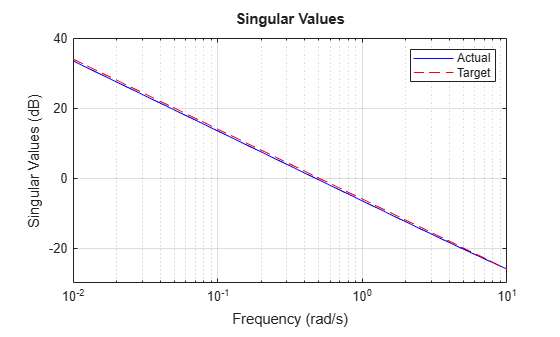Examine the step response of the closed-loop system.

`step(CL)`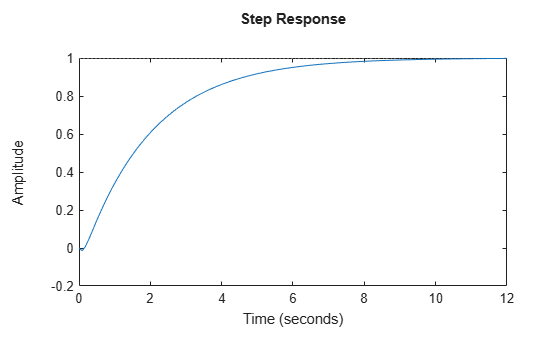You can use the input argument `alpha` to specify how much `loopsyn` favors either performance (`mixsyn` design) or robustness (`ncfsyn` design). By default, `loopsyn` computes a balanced design, `alpha` = 0.5. To change the balance, change `alpha`. Consider the following plant and target loop shape.

```G = tf(25,[1 10 10 10]); Gd = tf(0.5,[1 0]);```

Design a loop-shaping controller that maximizes performance (minimizes `gamma`) subject to the constraint that the robustness (as determined by `ncfmargin`) is no worse than 75% of the maximum achievable robustness. To do so, set `alpha` to 0.75.

```alpha = 0.75; [K,CL,gamma,info] = loopsyn(G,Gd,alpha);```

The maximum achievable robustness margin is returned in the `info` structure. Compare that value to the margin achieved by this controller. For `ncfmargin`, use the shaped plant and corresponding controller, also returned in `info`.

`info.emax`
```ans = 0.6474 ```
`ncfmargin(info.Gs,info.Ks)`
```ans = 0.4872 ```

These values confirm that the achieved robustness is 75% of the maximum robustness achievable by setting `alpha` = 1 for the pure `ncfsyn` design. For this plant, the `alpha` = 0.75 design yields a good match to the loop shape without sacrificing much robustness. Examine the performance and loop shape with this controller.

`gamma`
```gamma = 1.2150 ```
```sigma(G*K,"b",Gd,"r--",{0.01,10}) grid on legend("Actual","Target")```For details on how to choose a good value of `alpha` for your application, see Loop-Shaping Controller Design.

When designing a controller for a MIMO system, if you specify a scalar `Gd`, then `loopsyn` applies the same target loop shape to all feedback channels. You can specify a different shape for each loop using a diagonal `Gd` of size `Ny`-by-`Ny`, where `Ny` is the number of feedback loops, or the number of outputs of `G`. Consider the following control system with a two-output, two-input plant.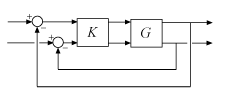```s = tf('s'); G = [(1+0.1*s)/(1+s) 0.1/(s+2) ; 0 (s+2)/(s^2+s+3)];```

Design a controller for this plant such as the first feedback channel has a crossover frequency of 1 rad/s and the second has a crossover frequency of 5 rad/s. To so, create the loop shapes `wc/s` for each loop and use the `append` command to create the diagonal `Gd`.

```wc = [1 5]; Gd = append(wc(1)/s,wc(2)/s);```

Design the controller.

```[K,CL] = loopsyn(G,Gd); ```

Compare the achieved loop shapes with the target loop shape.

```bodemag(G*K,Gd,{0.1,100}) grid on legend("Actual","Target")```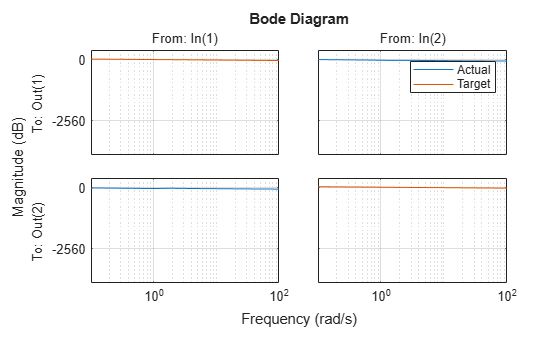You can limit the order of the controller that `loopsyn` designs using the `order` argument. To specify a controller order, you must use a value of the parameter `alpha` such that `0 < alpha < 1`.

```load plant_loopsynOrderExample.mat G size(G)```
```State-space model with 2 outputs, 2 inputs, and 7 states. ```

Design a controller for this plant with loop shape `Gd = 0.5/s`. Use `alpha = 0.5` and let `loopsyn` select the controller order.

```alpha = 0.5; Gd = tf(0.5,[1 0]); [K0,CL0,gamma0] = loopsyn(G,Gd,alpha); order(K0)```
```ans = 5 ```

`loopsyn` returns a controller with five states. Use `loopsyn` again to design a three-state controller.

```ord = 3; [K,CL,gamma,info] = loopsyn(G,Gd,alpha,ord); order(K)```
```ans = 3 ```

For this plant, reducing the controller order from five to three yields a small decrease in performance.

`gamma0`
```gamma0 = 1.0334 ```
`gamma`
```gamma = 1.1830 ```

Use a model-reduction command such as `balred` to identify suitable target orders to try for `ord`.

`loopsyn` can also provide a two-input, one output controller suitable for implementing the two-degree-of-freedom (2-DOF) architecture of the following illustration.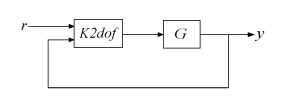This architecture can be useful for mitigating the derivative kick that can occur when the reference signal changes. To obtain a controller suitable for this implementation, specify a plant and target loop shape, and call `loopsyn`.

```G = tf(8625,[1 2.389 -5606]); Gd = tf(80,[1 0])*tf(240,[1 240]); [K,CL,gamma,info] = loopsyn(G,Gd);```

The `K2dof` field of the `info` output contains the 2-DOF controller. For a plant with `Nu` inputs and `Ny` outputs, `K2dof` has `Nu` outputs and `2*Ny` inputs. In this example, because `G` is SISO, `K2dof` has one output and two inputs.

```K2dof = info.K2dof; size(K2dof)```
```State-space model with 1 outputs, 2 inputs, and 4 states. ```

To use the controller, create a closed-loop system with the architecture shown previously.

```L2dof = G*K2dof; L2dof.InputName = {'r','y'}; L2dof.OutputName = 'y'; CL2dof = connect(L2dof,'r','y');```

Compare the closed-loop step response with the two architectures. For this system, the 2-dof architecture substantially reduces the overshoot in the response.

```step(CL,CL2dof) legend("1-dof","2-dof")```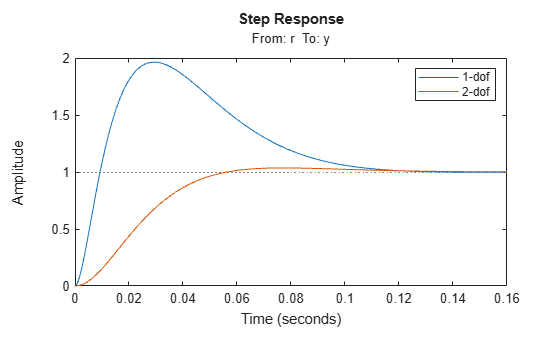## Input Arguments

collapse all

Plant, specified as a dynamic system model such as a state-space (`ss`) model. If `G` is a generalized state-space model with uncertain or tunable control design blocks, then `loopsyn` uses the nominal or current value of those elements. `G` can be SISO or MIMO, and can be a continuous-time or discrete-time model. `G` must have at least as many inputs as outputs. `G` cannot have time delays. Use `pade` to approximate delays.

Target loop shape, specified as a dynamic system model such as a `ss`, `tf`, or `zpk` model. You can also provide `Gd` as a frequency-response data (`frd`) model specifying the desired gain at specific frequencies. `Gd` cannot have time delays. Use `pade` to approximate delays.

For a MIMO plant `G` with `Ny` outputs:

• Specify SISO `Gd` to use the same desired loop shape for all loops.

• Specify `Ny`-by-`Ny` diagonal `Gd` to use a different shape for each feedback loop. One way to construct such a diagonal `Gd` is to specify a `Gdi` for each channel, and then use ```Gd = append(Gd1,...,GdNy)```.

In general, use a target loop shape that has high gain at low frequencies for reference tracking and disturbance rejection, and low gain at high frequencies for robustness against plant uncertainty. For more information about how to choose your target loop shape, see Loop Shaping for Performance and Robustness.

Balance between performance and robustness, specified as a scalar value in the range [0,1]. Use `alpha` to adjust the balance between performance and robustness as follows:

• `alpha = 0` gives the `mixsyn` design.

• `alpha = 1` gives the `ncfsyn` design.

`loopsyn` maximizes performance (minimizes `gamma`) subject to the constraint that the robustness (as measured by `ncfmargin`) is no worse than `alpha*emax`, where `emax` is the maximum robustness achievable by the `ncfsyn` design. The default value `alpha = 0.5` yields a balanced design. You can adjust `alpha` between 0 and 1 to find the right tradeoff for your application. For an example that shows the effect of varying `alpha`, see Loop-Shaping Controller Design.

Controller order, specified as a positive integer. To use this option, you must specify `alpha` such that `0 < ``alpha```` < 1```.

## Output Arguments

collapse all

Loop-shaping controller, returned as a state-space (`ss`) model. `K` shapes the open-loop response `G*K` to approximately match the specified loop shape `Gd`. The controller minimizes the performance `gamma` subject to the constraint that the stability margin as computed by `ncfmargin` does not exceed `alpha``*emax`, where `emax` is the maximum margin achievable by `ncfsyn`.

Closed-loop system, returned as a state-space (`ss`) model. The closed-loop system is given by `feedback(G*K,eye(ny))`, where `ny` is the number of outputs of `G`.

Controller performance, returned as a nonnegative scalar value or `Inf`. A value near or below 1 indicates that `G*K` is close to `Gd`. Values much greater than one indicate a poor match between the achieved and desired loop shapes. If `loopsyn` cannot find a stabilizing controller, `gamma` is `Inf`.

`gamma` is the mixed-sensitivity performance, the cost function minimized by `mixsyn`, and is given by

`$\gamma ={‖\left[\begin{array}{c}{W}_{1}S\\ {W}_{3}T\end{array}\right]‖}_{\infty },\text{ }S={\left(I+GK\right)}^{-1},\text{ }T=I-S,$`

where W1 and W3 are the `mixsyn` weights. `loopsyn` derives these weights from `Gd` to enforce the desired loop shape.

Additional information about the controller synthesis, returned as a structure containing the following fields.

FieldDescription
`W`Shaping prefilter, returned as a state-space (`ss`) model. The value of `W` is such that the shaped plant ```Gs = G*W``` has the desired loop shape `Gd`.
`Gs`Shaped plant `Gs = G*W`, returned as an `ss` model.
`Ks`H controller for the shaped plant `Gs` (see `ncfsyn`), returned as an `ss` model. For computing the robustness margin with `ncfmargin`, use `Gs` and `Ks`.
`emax`Maximum achievable robustness margin, returned as a scalar. This value is the robustness achieved by the pure `ncfsyn` design. For information on interpreting this value, see `ncfmargin`.
`W1,W3`Weighting functions for the `mixsyn` formulation of the loop-shaping goal, returned as `ss` models. `loopsyn` derives these weights from `Gd` to enforce the desired loop shape.
`K2dof`

Two-degree-of-freedom controller, returned as a `ss` model with `2*ny` inputs and `nu` outputs, where `ny` and `nu` are the numbers of outputs and inputs of `G`, respectively. This controller is suitable for a two-degree-of-freedom implementation that separates the reference signal from the feedback signal, as in the architecture of the following diagram.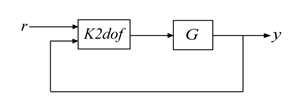For an example that shows how to use `K2dof`, see Two-Degree-of-Freedom Loop-Shaping Controller.

## Version History

Introduced before R2006a

expand all

Behavior changed in R2021b